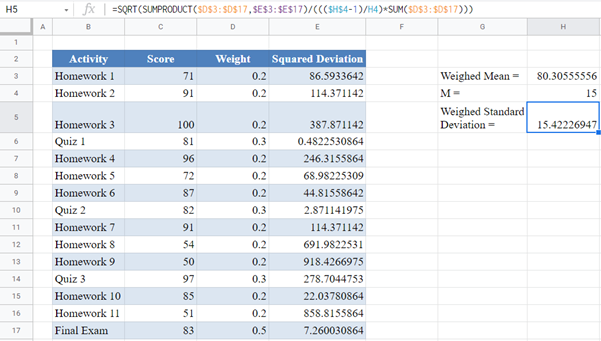# Weighted Standard Deviation – Excel and Google Sheets

This tutorial will demonstrate how to calculate weighted standard deviation in Excel and Google Sheets.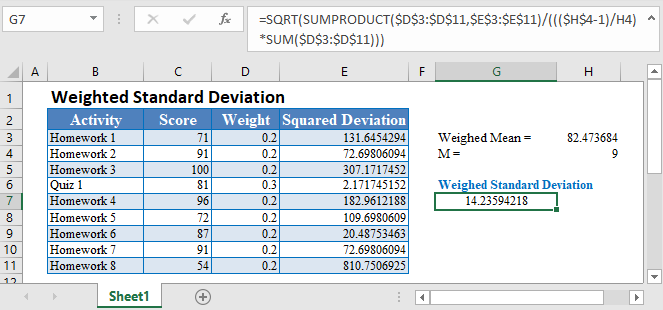Standard Deviation measure the spread of a data distribution. It measures the average distance of each data point from the mean of the dataset.Weighted Standard Deviation measures the spread of a data distribution from the mean where some of the data are more significant than others. The weighted standard deviation is calculated based on the weighted mean and it attaches more importance to data that have more weight than to data with less weight.

## Weighted Standard Deviation Formula

The weighted standard deviation is calculated using the formula: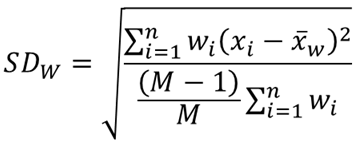where wi are the weights corresponding to each individual observation;

xi are the individual observations;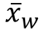is the weighted mean;

M is the number of non-zero weights;

Σ is a Greek letter called sigma which represents ‘sum’; and

n is the sample size (the number of observations).

## How to Calculate Weighted Standard Deviation in Excel

Background: Hilary is taking a college statistics course. The course is made up of 15 weeks of activities consisting of 11 homework, 3 quizzes, and a final exam. The final exam, the quizzes, and the homework contribute 50%, 30%, and 20% to the final grade respectively.  The table below shows the scores (out of 100) Hilary earned in each of the activities. Calculate the weighted standard deviation of Hilary’s scores.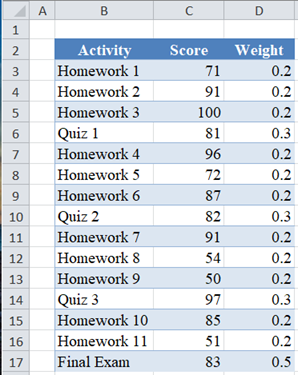Next, calculate the Weighted Mean using the SUMPRODUCT Function.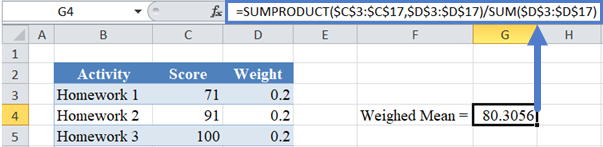Next, calculate the Squared Deviation of the scores from the Weighted Mean.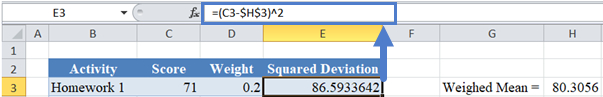Note: Double-Click the bottom right corner of the cell to fill down the data to the rest of the column.

The complete Squared Deviation column is shown below.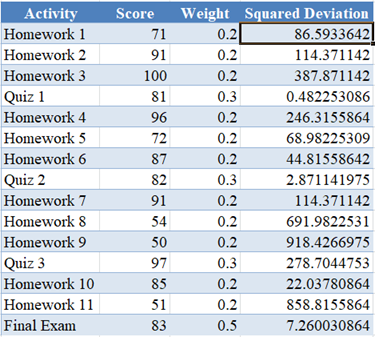Next, obtain M, the number of non-zero weights.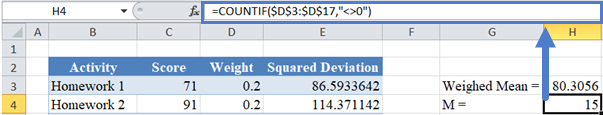Note: The symbol  represents the ‘not equal to’ operator in Excel.

Finally, calculate the Weighed Standard Deviation using the SUMPRODUCT Function as shown below: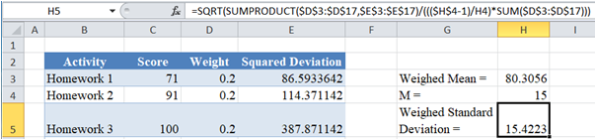## Weighted Standard Deviation in Google Sheets

The Weighted Standard Deviation can be calculated in Google Sheets in the exact same way as it is calculated in Excel as shown in the picture below.